# CalcPro

carlosgs Download

## Description

This is an engineering calculator, with equation solvers, interpolations, matrices, wolfram alpha, converter, complex numbers, custom functions, variables... and more in the future!

This is an application designed for engineers but can be used by anyone. Once you open the app you'll see a simple calculator with a swipe keyboard but there's much more behind.

NOTE: SWIPE THE KEYBOARD TO SEE ALL THE FEATURES

Landscape mode plays nicely with your tablet. Most of the features are just a tap away now.

This is a powerful application which has the following features:

- Equation Solver: You can solve almost any equation. It supports:
-> Non-linear equations: Any type of equation, such as: 5*x+sin(x^3+2)=8 (up to 10 equations and variables, you can see 2 equations solved in the screenshots)
-> Linear equations: Like 2*x+3*y=8, -3*x+6*y=3 (up to 10)
-> Polynomials: Up to grade 20 (complex and non-complex solutions)

- Interpolation
-> Simple: Perfect for thermodynamic tables with only one variable (for example Temperature)
-> Double: 2 variables interpolation. See screenshots for a preview

- Curve fitting
-> You can get the closest curve given a set of points. You can choose between: linear, parabola, 3-degree polynomial, power, exponential, logarithmic or even create your own curve. The tool will calculate square R too.

- Matrices: Since version 1.3 you can use matrices. Matrices are made of complex and real numbers and contain most common operations. Functions and operators currently supported: +, -, *, /, determinant, transpose, ^,.^,.*,./, ^-1 (Inverse), get, set, eyes (Identity matrix), ones. You can sum a number to a matrix similarly to Matlab, power each element individually...

- Vectors: Complex/real vectors with dot and cross multiplication (besides the applicable methods found in matrices)

- Function editor: Create as many functions as you want and save them under different categories. Using custom functions is extremely easy and very helpful. You can describe the functions and variables. Furthermore, you can take a photo that may help you understand and use the function correctly. Functions can be used in the calculator as well as in the equation solver.

- 2D function plotter (Still in beta)

- Converter: It's useful to have a converter inside the app. It's got 19 conversions: length, area, volume, angle, time, speed, acceleration, flow, mass, force, torque, energy, density, pressure, dynamic viscosity, kinematic viscosity, temperature and currency.

- Wolfram Alpha search: Just touch the search button

- Complex numbers: The calculator supports complex numbers in both rectangular and polar notation. Polar notation us useful for phasors in Circuit Analysis.

- Variables: Just save any number as a variable and use it later.

- Constants: 6, more in next updates.

- Radians/degrees

- Easier decimal notation: 1.000000 is 1, 99999999 is 9.999E7 (depending of the decimal precision)...

- And probably more features in the future!

Permissions explained:
- INTERNET: To browse WolframAlpha
- WRITE_EXTERNAL_STORAGE: To store custom function photos in the SD card if available

This application can be moved to the SD card so it only takes about 1.3MB of Internal Storage.

## Screenshots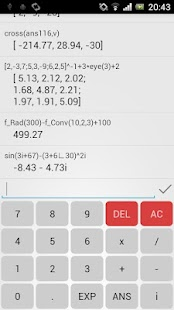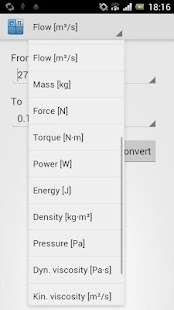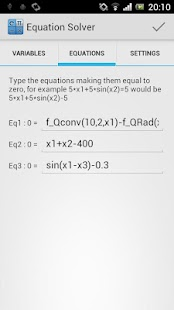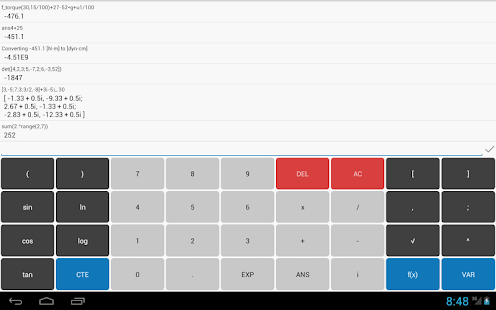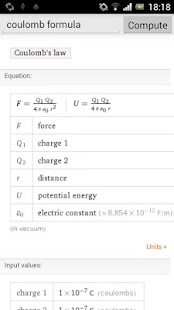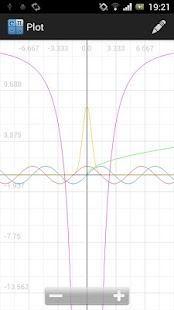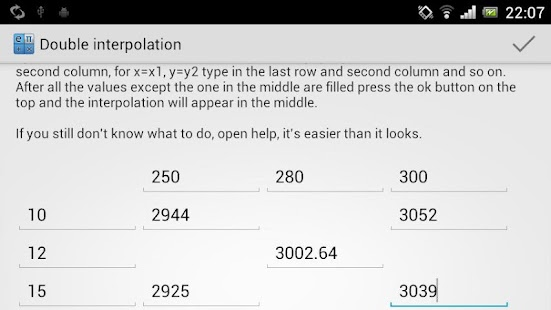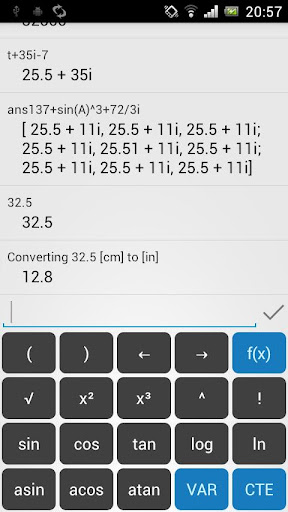## Downloads

Download cracked APK of CalcPro ( com.carlosgs91.calcpro )

## Comments

No Comments yet.
You have to login
27547 Apps online